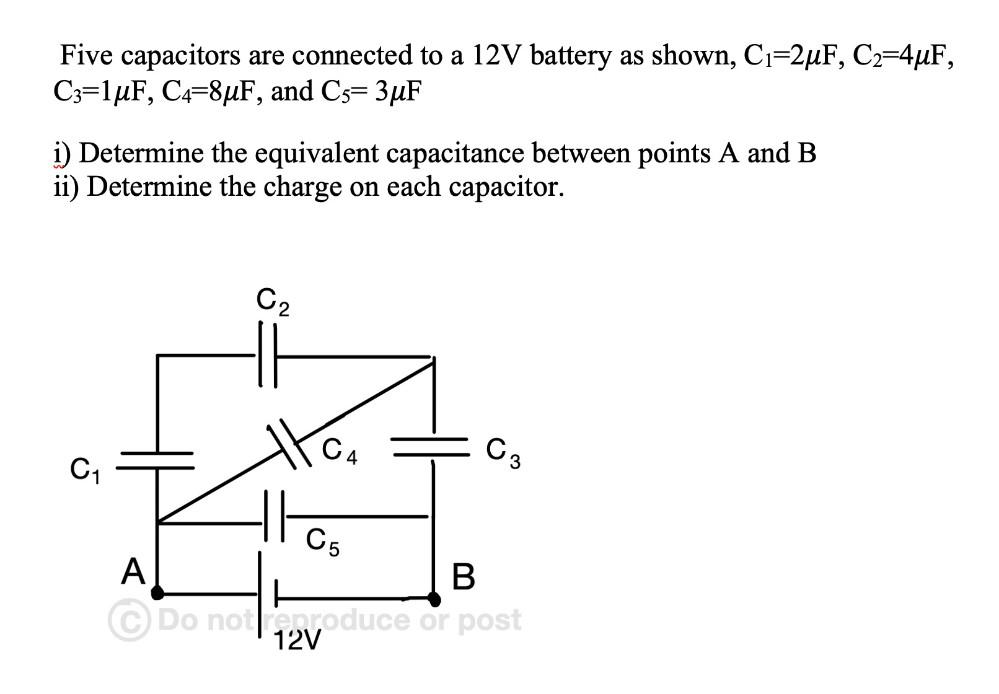Question:

# Five capacitors are connected to a 12V battery as shown, C=2uF, C2=44F, C3=1uF, C4=8uF, and C5= 3uF i) Determine the equivalentFive capacitors are connected to a 12V battery as shown, C=2uF, C2=44F, C3=1uF, C4=8uF, and C5= 3uF i) Determine the equivalent capacitance between points A and B ii) Determine the charge on each capacitor. C2 C C3 C1 TH C5 A B © Do not reproduce or post